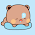Tuesday, February 26, 2013

Prime Factorization and Divisor Function σx(n)

σx(n) is defined as the sum of xth powers of the distinct positive divisors of n. The function can be expressed as:

Here, r = ω(n), which is the number of distinct positive prime factors of n. pi for i = 1 to r, are the prime factors and ai is the maximum power of piby which n is divisible.

Clearly, this function can be used for various problems, for example, when x = 0, it simply means the number of distinct positive divisors of n, if x = 1, it is the sum of distinct positive divisors of n, for x = 2, its the sum of squares of positive divisors of n and so on.

For programming contest practice, there are a few problems that requires sum of divisors, or number of divisors, which can be calculated by simply calculating the prime factors with their count, and then evaluating the function shown above with appropriate value on x. Also, the form of function definition can be changed when you set a value for x. After putting x = 0, we get this:

So this is easy, you just need to find frequency of each prime, and then multiply each (ai + 1) for all primes, you get the number of divisors of n.

For sum of divisor, the idea is similar, here x = 1. The following code shows how to find sum of distinct positive divisors of n:

```#include <cstdio>
#include <cmath>
using namespace std;

#define sq(x) ((x)*(x))
#define i64 unsigned long long
#define MAX 784
#define LMT 28

unsigned flag[MAX/64];
unsigned primes, total;

#define chkC(n) (flag[n>>6]&(1<<((n>>1)&31)))
#define setC(n) (flag[n>>6]|=(1<<((n>>1)&31)))

/*
Regular sieve of eratosthenes, bitwise implementation
*/
void sieve()
{
unsigned i, j, k;
flag|=0;
for(i=3;i<LMT;i+=2)
if(!chkC(i))
for(j=i*i,k=i<<1;j<MAX;j+=k)
setC(j);
primes[(j=0)++] = 2;
for(i=3;i<MAX;i+=2)
if(!chkC(i))
primes[j++] = i;
total = j;
}

/*
finds n^p in log(p) time
*/
i64 power(unsigned n, unsigned p)
{
i64 x=1, y=n;
while(p > 0)
{
if(p&1) x *= y;
y *= y;
p >>= 1;
}
return x;
}

/*
calculates the sigma(1) function, we don't need to find prime frequencies.
*/
inline void update(i64 &sigma1, i64 n, unsigned p)
{
if(p==1) sigma1 *= (n+1);
else sigma1 *= ((power(n,p+1)-1)/(n-1));
}

/*
Factorization function, we do not need to store the primes here,
instead, whenever a prime is found, you update corresponding prime
and frequency with sigma 1
*/
void factor(i64 n, i64 &sigma1)
{
unsigned i, v;
i64 t;
for(i=0, t=primes[i]; i<total && t*t <= n; t = primes[++i])
{
if(n % t == 0)
{
v = 0;
while(n % t == 0)
{
v++;
n /= t;
}
update(sigma1, primes[i], v);
}
}
if(n>1) update(sigma1, n, 1);
}

/*
Our beloved main function
*/
int main()
{
int t, x;
i64 n, sigma1;
sieve();
scanf("%d", &t);
for(x=1; x<=t; x++)
{
scanf("%llu", &n);
factor(n, sigma1);
printf("%llu\n",sigma1);
}
return 0;
}
```

We just need the values of σ, so here we will not store the prime factors. Some similar problem would be to find the number of odd divisors of n, or testing if a number is square free, i.e. no perfect square divides n, going to leave these as an exercise for readers.

1.1.